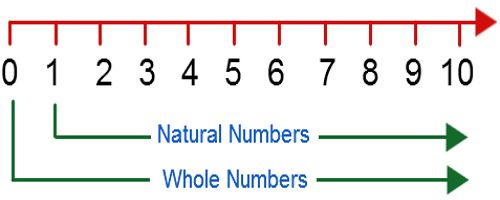A Whole Number is a number that is not a fractional number. Whole Numbers are simply the numbers 0, 1, 2, 3, 4, 5, … (and so on). There is no fractional or decimal part and no negatives. It is a number that is not a fraction of a number, a percentage or has a decimal.

Examples of Whole Numbers

Some examples of whole numbers are 4, 5, and 89,453,711.

Any fraction, decimal, negative number or imaginary number is not a whole number. For example, -5, 6.788, and 4/5 are not whole numbers.

The Set of Whole Numbers

The set of whole numbers is closed under addition and multiplication. What that means is that for all whole numbers a and b, a + b = c and a b = d will also be whole numbers.

Just as 6 + 7 = 13 and 6 · 7 = 42 are whole numbers, the sum or product of any two whole numbers will also be whole.

The set of whole numbers isn’t closed under division and subtraction. For any whole number a, there is another whole number b such that a – b = c and a / b = d are not whole numbers.

Example: 7 and 9 are whole numbers, but 7 – 9 = -2, which is not a whole number. 4 and 5 are whole numbers, but 4/5 is not a whole number.Properties of Whole numbers

Whole numbers are commutative under addition and multiplication . For every a, b in the set of whole numbers, a + b = b + a and a · b = b a.

Whole numbers are associative under addition and multiplication. For every a, b, and c in the set of whole numbers, a(b·c) = (a·b) · c and (a + b) + c = a + (b + c).

The set of whole numbers includes an additive identity 0 so that a + 0 = a for every whole number a. It also includes a multiplicative identity 1 so that 1 · a = a.

Distributive Property: If x,y and z are three whole numbers, the distributive property of multiplication over addition is x*(y+z)=(x*y)+(x*z), similarly the distributive property of multiplication over subtraction is x*(y-z)=(x*y)-(x*z)

Multiplication by zero: When a whole number is multiplied to 0, the result is always 0, i.e., x*0=0*x=0

Division by zero: Division of a whole number by o is not defined, i.e., if x is a whole number then x/0 is not defined.

Statistics how:

Statistics How